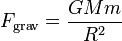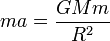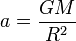# Acceleration due to gravity

## Definition

Acceleration due to gravity refers to the value of the acceleration on one body due to another that would arise as a result of the gravitational force between the bodies, if there were no other forces on that body. In other words, that body is in free fall.

### The case of the earth or a large planet

Consider the earth and an object near the surface of the earth, so that, for practical purposes, the distance between their centers of mass can be taken to be the radius of the earth. If$M$ is the mass of the earth,$R$ is the radius of the earth, and$m$ is the mass of this other object, then the magnitude of gravitational force exerted by the earth on the object is:$F_{\operatorname{grav}} = \frac{GMm}{R^2}$

where$G$ is the gravitational constant. If the body with mass$m$ were in free fall, Newton's second law of motion would yield:$ma = \frac{GMm}{R^2}$

where$a$ is the acceleration of the body in the direction of the center of the earth (i.e., downward). We thus get:$a = \frac{GM}{R^2}$

Note that the quantity on the right side is independent of$m$, and this quantity is called the acceleration due to gravity, and is denoted by the letter$g$. We thus get:$g = \frac{GM}{R^2}$